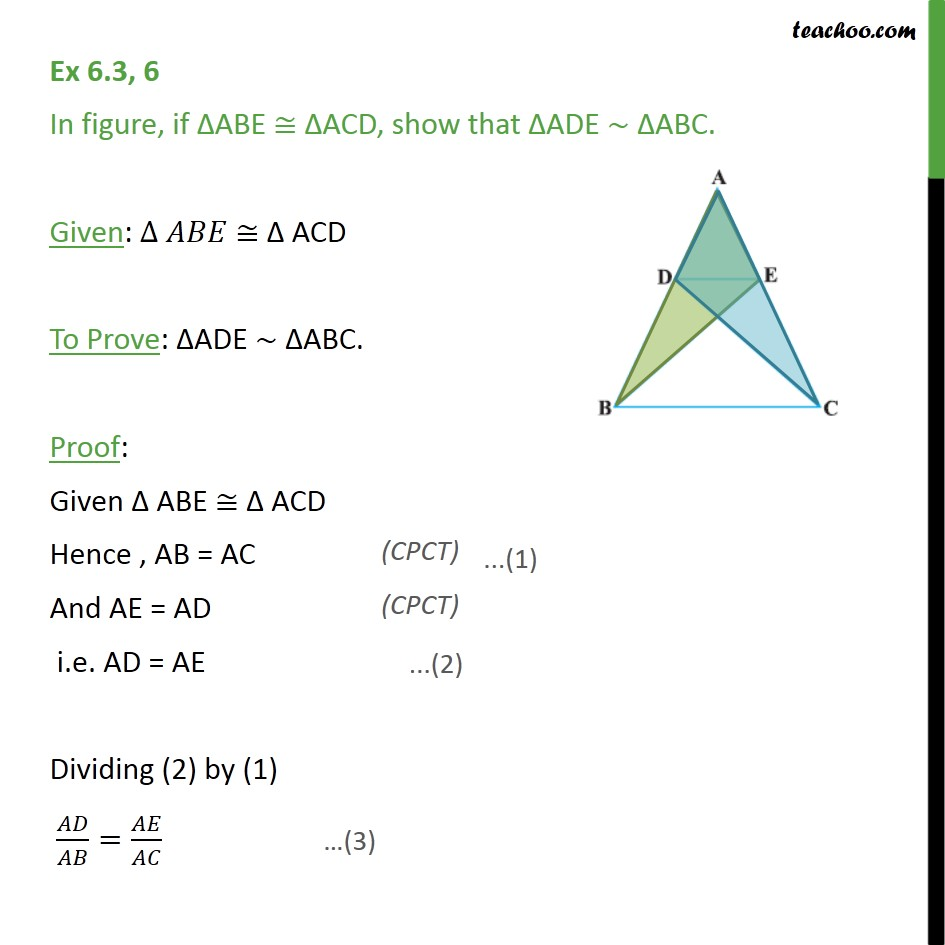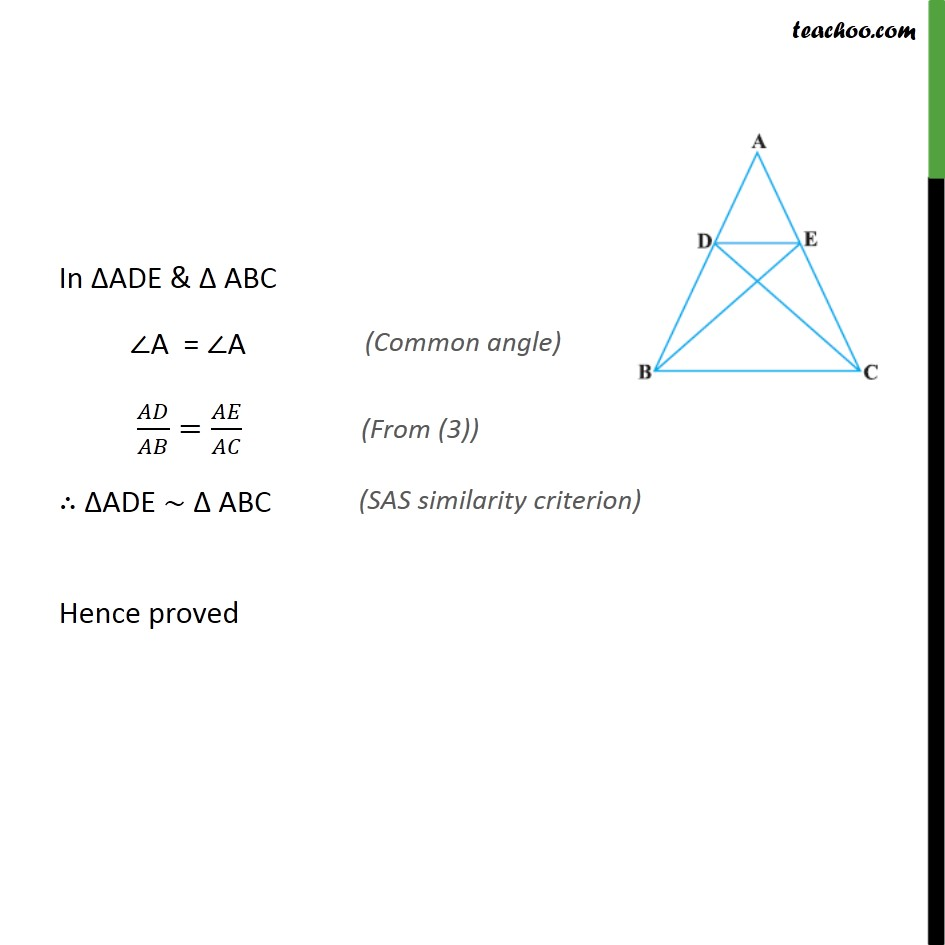1. Chapter 6 Class 10 Triangles
2. Serial order wise
3. Ex 6.3

Transcript

Ex 6.3, 6 In figure, if ABE ACD, show that ADE ABC. Given: ACD To Prove: ADE ABC. Proof: Given ABE ACD Hence , AB = AC And AE = AD i.e. AD = AE Dividing (2) by (1) / = / In ADE & ABC A = A / = / ADE ABC Hence proved

Ex 6.3

Chapter 6 Class 10 Triangles
Serial order wise